Next: Power Spectrum Analysis using Up: Analysis of the Data Previous: Analysis of the Data

## Normal ACF analysis using a Phase Fit

The problem of determining the mean Doppler velocity, assuming there is only one velocity component in the scattering area, can be solved using a very simple approach. The radar interpreter produces a complex autocovariance function A given by: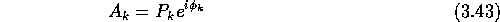whereis the amplitude at a given lag, k the lag index and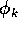the phase at a given lag. The phase of the complex value as a function of lag is determined by the Doppler velocity spectrum. For a system with a single velocity component, the phase will change linearly with lag time. The phase increases for positive velocities (toward the radar) and decreases for negative velocities (away from the radar). Therefore a simple least squares fit of the phase of the ACF as a function of time will result in a straight line which passes through 0 at lag 0. The slope of this line corresponds to the mean Doppler frequency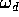, which can be used to determine the Doppler velocity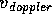. The problem with this method is that the measurements can be used to calculate the phaseonly within the range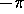to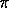(the residual phase,), but for large velocities the phase change over the entire ACF can easily exceed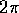. There exists a unique and correct solution which can be found through various methods. Using a ``brute-force'' method, one can minimize the error function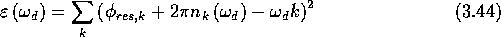whereis the measured residual phase at lag k,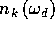is an integer calculated for each lag andis, as before, the Doppler shift frequency. This calculation can be performed for a number of differentvalues until a minimum is determined. Thecorresponding to this minimum is taken to be the desired Doppler frequency. The amount of processing required for the brute-force solution is relatively highand a different technique is employed in the actual program. It involves calculating a first guess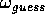using one or more close pairs of residual phases and then adjustinguntil a minimum error is found. The Doppler frequency(which can be negative) corresponds to the mean Doppler velocity and can be converted to the mean line-of-sight component of the plasma drift velocity using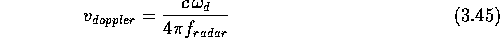where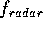is the radar transmission frequency and the assumption is made that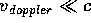.

The backscattered power and the spectral width are parameters that can be determined from the ACF once the Doppler velocity is known. The amplitude variation of the ACF is independent of the phase variation. If the scattered signal has a spectrum S which is Lorentzian in shape, that is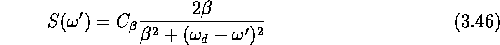where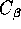is the maximum of the associated autocovariance function and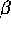a constant, then the amplitude variation of the ACF is well represented by an exponential decay. This is usually the case [HVG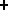93]. There are two exceptions to this rule. Very narrow spectra observed in the E-region often appear to be more characteristic of a Gaussian shape [Han91], while spectra in the cusp region are broad and typified by multiple Gaussian components. Disregarding these exceptions, the amplitude variation of the ACF can be written as the exponential form:where C is a maximum backscattered power,becomes a decay constant, k is the lag index and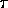the basic lag separation time. A linear least-squares fit to the measured values of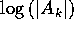can be used to determine numerical values for C and. Since the autocovariance function and the spectral density function constitute a Fourier transform pair, we can determine the relationship of these values to the half-power spectral width of the power spectrum and arrive at a formula for the Doppler velocity width: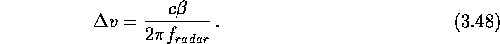This follows because the full width at half maximum is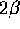.

The method described here is computationally not expensive and is well adapted to a situation where the Doppler power spectrum is dominated by a single component. Although it is used for all calculations on all radars, it has a number of shortcomings [Bak90], as follows.

• The method cannot handle multiple Doppler components in general. It does not give any indication of the presence of multiple Doppler velocities in the target volume and will return wrong results for this case.
• The progressive-fit method employed for the actual (real-time) calculation of the velocity can produce wrong results if a local minimum in the error function is found rather than the global minimum.
• The power and width estimates are based on the assumption of an exponential amplitude decay; this assumption is wrong in certain cases.

The validity of the current method has been checked in several studies involving the comparison between different techniques. Grant et al. [GMR95] arrive at a variational difference of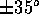in direction and% in speed with no systematic difference, when comparing SuperDARN drift velocities with measurements from the Canadian Advanced Digital Ionosonde (CADI).Next: Power Spectrum Analysis using Up: Analysis of the Data Previous: Analysis of the Data

Andreas Schiffler
Wed Oct 9 10:05:17 CST 1996